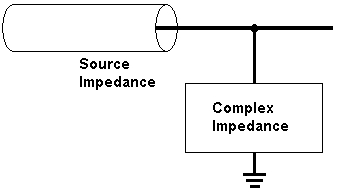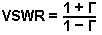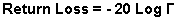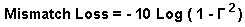# Return Loss and Mismatch Loss Calculator

Calculates the absolute load impedance, reflection coefficient, VSWR, return loss and mismatch loss of a load.Enter the source characteristic impedance and the load impedance then press "Calculate" below.

 INPUT DATA Source Impedance: Ohms Load Impedance R: Ohms Load Impedance J: Ohms

This calculator uses JavaScript and will function in most modern browsers. For more information see About our calculators.

The absolute value of the load impedance is calculated from the complex impedance (R + j).Where R is the Real part of the impedance

and j is the Imaginary part of the impedance

The Load Reflection Coefficient ( Γ ) is calculated using the complex impedance of the load and the characteristic impedance of the source.Where Zo is the Source Impedance

The VSWR is then calculated using the Reflection CoefficientThe Reflection Coefficient is used again to calculate the Return LossThe Reflection Coefficient is used yet again to calculate the Mismatch LossVarious equations for Voltage Reflection Coefficient and VSWR are also available at:-

http://www.spectrum-soft.com/news/fall2009/vswr.shtm

This calculator is provided free by Chemandy Electronics in order to promote the FLEXI-BOX